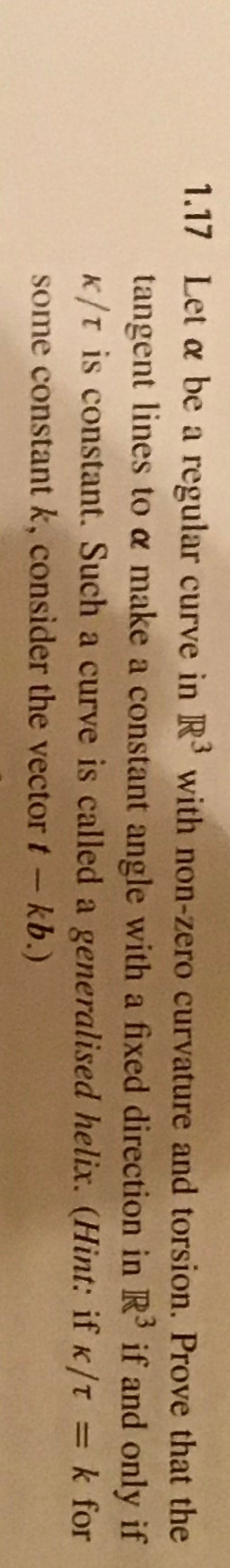Home / Answered Questions / Other / 1-17-let-a-be-a-regular-curve-in-r-with-non-zero-curvature-and-torsion-prove-that-the-tangent-lines--aw788

# (Solved): 1.17 Let A Be A Regular Curve In R With Non-zero Curvature And Torsion. Prove That The Tangent Lines...1.17 Let a be a regular curve in R with non-zero curvature and torsion. Prove that the tangent lines to a make a constant angle with a fixed direction in R if and only if K/t is constant. Such a curve is called a generalised helix. (Hint: if k/t = k for some constant k, consider the vector t - kb.)

We have an Answer from Expert